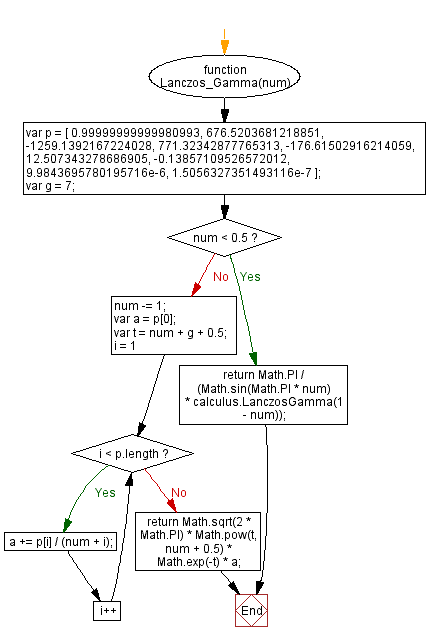# JavaScript: Calculate Lanczos approximation gamma

## JavaScript Math: Exercise-49 with Solution

Write a JavaScript function to calculate Lanczos approximation gamma.

In mathematics, the Lanczos approximation is a method for computing the Gamma function numerically, published by Cornelius Lanczos in 1964. It is a practical alternative to the more popular Stirling's approximation for calculating the Gamma function with fixed precision.

Sample Solution:-

HTML Code:

``````<!DOCTYPE html>
<html>
<meta charset="utf-8">
<title>JavaScript function to calculate Lanczos approximation gamma</title>
<body>

</body>
</html>
```
```

JavaScript Code:

``````function Lanczos_Gamma(num)
{
var p = [
0.99999999999980993, 676.5203681218851, -1259.1392167224028,
771.32342877765313, -176.61502916214059, 12.507343278686905, -0.13857109526572012, 9.9843695780195716e-6, 1.5056327351493116e-7
];
var i;
var g = 7;
if (num < 0.5) return Math.PI / (Math.sin(Math.PI * num) * calculus.LanczosGamma(1 - num));
num -= 1;
var a = p;
var t = num + g + 0.5;
for (i = 1; i < p.length; i++) {
a += p[i] / (num + i);
}
return Math.sqrt(2 * Math.PI) * Math.pow(t, num + 0.5) * Math.exp(-t) * a;
}
console.log(Lanczos_Gamma(5));
```
```

Sample Output:

```23.999999999999996
```

Flowchart:Live Demo:

See the Pen javascript-math-exercise-49 by w3resource (@w3resource) on CodePen.

Improve this sample solution and post your code through Disqus

What is the difficulty level of this exercise?

﻿

## JavaScript: Tips of the Day

What is the !! (not not) operator in JavaScript?

Converts Object to boolean. If it was falsey (e.g. 0, null, undefined, etc.), it will be false, otherwise, true.

```!oObject  // inverted boolean
!!oObject // non inverted boolean so true boolean representation```

So !! is not an operator, it's just the ! operator twice.

Real World Example "Test IE version":

```const isIE8 = !! navigator.userAgent.match(/MSIE 8.0/);
console.log(isIE8); // returns true or false
```

If you =>

```console.log(navigator.userAgent.match(/MSIE 8.0/));
// returns either an Array or null```

But if you =>

```console.log(!!navigator.userAgent.match(/MSIE 8.0/));
// returns either true or false
```

Ref: https://bit.ly/3ezKiqB